## research papersJOURNAL OFSYNCHROTRONRADIATION
ISSN: 1600-5775
Volume 25| Part 6| November 2018| Pages 1841-1846

## Feasibility study of phase-contrast X-ray lamino­graphy using X-ray interferometry

aSAGA Light Source, 8-7 Yayoigaoka, Tosu, Saga 841-0005, Japan, bResearch and Development Group, Hitachi Ltd, 2520 Akanuma, Hatoyama, Saitama 350-0395, Japan, cInstitute of Materials Structure Science, High Energy Accelerator Research Organization, 1-1 Oho, Tsukuba, Ibaraki 305-0801, Japan, dResearch and Development Group, Hitachi Ltd, 1-280 Higashi-koigakubo, Kokubunji, Tokyo 185-8601, Japan, eResearch Institute for Material and Chemical Measurement, National Institute of Advanced Industrial Science and Technology (AIST), Central 5, 1-1-1 Higashi, Tsukuba, Ibaraki 305-8565, Japan, and fSchool of Allied Health Sciences, Kitasato University, 1-15-1 Kitasato, Minami-ku, Sagamihara, Kanagawa 252-0373, Japan
*Correspondence e-mail: yoneyama@saga-ls.jp

(Received 4 June 2018; accepted 29 September 2018; online 25 October 2018)

For fine observation of laminar samples, phase-contrast X-ray lamino­graphy using X-ray interferometry was developed. An imaging system fitted with a two-crystal X-ray interferometer was used to perform the observations, and the sectional images were calculated by a three-dimensional iterative reconstruction method. Obtained images of an old flat slab of limestone from the Carnic Alps depicted fusulinids in the Carboniferous period with 3 mg cm−3 density resolution, and those of carbon paper used for a fuel-cell battery displayed the inner fibrous structures clearly.

### 1. Introduction

X-ray computed tomography is widely used for non-destructive three-dimensional observation of the inner structure of samples in various fields. For observation of laminar samples such as paper and printed circuit boards, high-energy X-rays and/or long exposure times are required to obtain a transmitted image at the rotational angle where the long edge of the sample is parallel to the X-ray direction. As a result, the density resolution was decreased and fine images could not be obtained. To overcome this limitation, computed lamino­graphy has recently been developed (Gondrom et al., 1999; Helfen et al., 2005). By inclining the sample rotational axis from the perpendicular axis of the X-rays, the path length in the sample is decreased and becomes constant so the X-rays can transmit the sample at any rotational angle and sectional images can be easily obtained. Various observations of fossils (Houssaye et al., 2011) and semiconductors (Helfen et al., 2011) were performed successfully using synchrotron radiation. In addition, phase-contrast lamino­graphy, which depicts X-ray phase shifts passing through a sample, was also developed. For light elements, since the cross section of the phase shift is about 1000 times larger than that of absorption in the hard X-ray region, the sensitivity is much higher than that of conventional absorption-contrast imaging (Momose & Fukuda, 1995). Fine observations of fuel-cell diffusion layers and paper were demonstrated using a propagation method (Helfen et al., 2009), Talbot interferometry (Harasse et al., 2011) and the diffraction enhanced method (Hirano et al., 2016). However, the density resolution is estimated to be around tens of mg cm−3 and therefore fine observation of samples with small density differences could not be performed. Furthermore, the X-ray energy is below ∼20 keV and thick samples could also not be observed.

The sensitivity of crystal X-ray interferometric imaging is highest among phase-sensitive methods such as diffraction enhanced imaging (DEI) and Talbot interferometric imaging (Pagot et al., 2005; Diemoz et al., 2012; Zhou et al., 2013; Yoneyama et al., 2008, 2015). This is because the method directly detects the phase shift by the superposition of the waves while other methods detect the spatial gradient of the phase shift. As a result of this advantage, X-ray interferometric imaging has the highest density resolution and provides a way to perform fine observations of biomedical and organic samples without using any supplemental methods (Momose et al., 1996). We have been developing a large-area imaging system fitted with a two-crystal X-ray interferometer using synchrotron radiation. In the first version of the imaging system, we designed new positional tables to attain the mechanical stability (∼prad) required for the operation of the interferometer and successfully generated a fine X-ray interference pattern (Yoneyama et al., 1999). In the second version, we expanded the field of view, adding an active feedback system to suppress long-term drift rotation, and performed phase-contrast computed tomography (CT) (Yoneyama et al., 2002). The third-generation system is currently in operation at beamline BL14C of the Photon Factory in Japan (Yoneyama et al., 2004; Yoneyama, Nambu et al., 2013), and many fine visualizations of β-amyloid plaques in brains taken from mice with Alzheimer's disease (Noda-Saita et al., 2006), energy materials such as methane (natural gas) hydrate (Takeya et al., 2011) and carbon paper used for fuel-cell batteries (Takeya et al., 2010) have been performed.

A combination of lamino­graphy and X-ray interferometry is expected to provide a method for us to visualize smaller density differences in laminar samples. In addition, high-energy X-rays can be used in X-ray interferometry with this system (Yoneyama et al., 2005), so it becomes possible to observe thick laminar samples. In this article, we will report on the principles of lamino­graphy, using an iterative reconstruction method, a third-generation imaging system and the obtained images of an old flat slab of limestone and carbon paper used for fuel cells.

### 2. Imaging system and reconstruction method

#### 2.1. Imaging system for lamino­graphy using a two-crystal X-ray interferometer

Fig. 1shows a schematic view of a two-crystal X-ray interferometer (Fig. 1a), an arrangement of sample and rotational axes for conventional CT (Fig. 1b) and a lamino­graphy arrangement (Fig. 1c). The incident X-rays were divided into two beams [object (O) and reference (R) beam] at the S wafer by Laue-case X-ray diffraction. Each beam is reflected by the M1 and M2 wafer, and the beams are recombined at the A wafer to generate two interference beams. By putting the sample in the O-beam path, the phase was shifted and the interference pattern was changed. Therefore, the phase shift caused by the sample could be detected by changes in the interference pattern. So far, thick samples could not be observed correctly because the interference-pattern fringes were too narrow, and the correct phase shift was undetectable (the phase-unwrap problem). Therefore, a fine phase map (spatial distribution of the phase shift) can be obtained with laminar samples at the facing angle, but three-dimensional observation by CT (Fig. 1b) could not be performed because the angle whereby the sample became thick could not be avoided.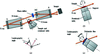Figure 1 (a) Schematic view of a two-crystal X-ray interferometer, (b) sample rotational arrangement for conventional CT and (c) a laminography arrangement.

To overcome the problem, the sample was rotated around the inclined axis from the CT axis for lamino­graphy in this study (Fig. 1c). The inclined angle is called the lamino­graphy angle and is normally set at 30–45°. By inclining the axis, the optical path in the sample is decreased and becomes constant in the central area of the sample, independent of the sample rotational angle. Therefore, the phase shift can be detected correctly, and the fine observation of the phase map can be performed by X-ray interferometry even with a narrow dynamic range.

#### 2.2. Reconstruction method

Since the X-ray is a parallel beam in the system, the sectional images can be obtained using a normal reconstruction algorithm such as the filtered-back projection (FBP) method for CT. On the other hand, a three-dimensional reconstruction method is required for lamino­graphy because the position of the sample in relation to the X-ray path changes depending on the sample rotational angle. Note that the obtained phase maps show the real part of the complex refractive index whereas the normal absorption-contrast image shows the imaginary part, so the phase map can be dealt with in the same way as absorption-contrast images for the reconstruction calculation.

The phase shift pθ(x, y) at the sample rotational angle θ is given by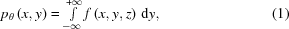where f is the phase shift at position (x, y, z) in the sample. The position can be written in the (xr, yr, zr) coordinate system using θ and the lamino­graphy angle φ,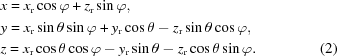Therefore, equation (1)becomes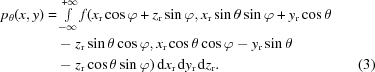Although the position depends on φ, pθ can be expressed as the addition of f, which is similar to normal CT. Therefore, the sectional images of lamino­graphy can be calculated by conventional reconstruction methods extended to three-dimensional treatment.

Two major reconstruction methods, a single method such as FBP and an iterative method, are mainly used for normal CT. The former method reconstructs the sectional image by one convolution of a filter and back-projection. The calculation procedure is simple and the computational complexity is small, so this method is mainly used for commercial CT systems. However, optimized filters for each sample are required, and known information about the sample cannot be used. The latter method reconstructs images by iterative calculation of the projection and the back-projection to reduce the error between the obtained and calculated images. The computational complexity and computational time are increased, but a filter is not required and known information about the sample can be used. Lamino­graphy is a kind of incomplete-data CT, so the decrease in the image quality was worrying. To overcome this problem, an iterative method was used to improve the image quality by using known information about the sample (i.e. whether the density is non-negative and the approximate shape).

An iterative reconstruction calculation based on the Jacobi method was performed using the following procedure:

(i) Initialize the iteration number (k = 0) and the iteration three-dimensional image [I(k) = 0].

(ii) Calculate the initial three-dimensional image Is by using a back projection of the obtained projection images Ip.

(iii) Calculate the (k + 1)-th correction three-dimensional image q(k + 1) by using projection and back projection of I(k).

(iv) Calculate the (k + 1)-th iteration three-dimensional image I(k + 1) using I(k + 1) = I(k) + 2[Isq(k + 1)].

(v) Check the CT value of each pixel of I(k + 1) and set it to 0 or the maximum if less than 0 or more than the maximum, respectively.

(vi) Set the CT value of I(k + 1) outside the sample to 0 or less depending on the sample.

(vii) Calculate the residual error dI from I(k) and Is [dI = |IsI(k + 1)|].

(viii) Increment k.

(ix) Repeat the procedure from (iii) to (viii) until dI is less than the convergence value or k reaches the set maximum iteration number.

A large number of the three-dimensional projections and back-projections are required to be processed with this method, so we used a GPU (general purpose unit), which calculates the projection and back-projection in parallel processes to shorten the calculation time. Before applying the algorithm to a real data set, we compared the reconstructed images of demonstrative data obtained by using normal (FBP) and iterative methods. The results show that the images of FBP contain many structures of different slices in the outer area and the original structure cannot be clearly distinguished. On the other hand, images of the iterative method do not contain other structures and the original structure was clearly visualized.

### 3. Results

The feasibility test was performed using the third-generation imaging system. The synchrotron radiation emitted from the vertical wiggler was monochromated at 35 keV using a two-crystal monochromator, enlarged horizontally five times by an asymmetric crystal and then irradiated onto the interferometer. One interference image was taken by a fiber-coupled large-area fast X-ray imager (Photonic Science, XDI-VHR60mm), which has a 53 mm × 35 mm field of view with a 12.5 µm pixel size. The phase map was obtained by a three-step fringe scanning method using the phase shifter shown in Fig. 1. The sample was rotated over 360° in 0.72° steps (500 projections). The exposure time for obtaining each interference pattern was set at 5 s and the total measurement time was around 3 h.

Decreasing the lamino­graphy angle can increase the spatial resolution in the y direction because the measurement becomes similar to that of normal CT. On the other hand, a small angle may cause a phase-unwrapping problem. Therefore, we optimized the lamino­graphy angle of each sample by the following procedure: (i) obtain a phase-map of the sample at a lamino­graphy angle from 20 to 60° in 5° increments, (ii) unwrap each obtained phase map and (iii) select the lowest angle in which the unwrapping was performed correctly. As a result, we set the angle at 30° for the flat slab of limestone and 45° for the carbon paper in this study. To perform a stabilized observation, each sample was attached to a thin polyimide film larger than the sample, and the film was attached to the top of the tube having a large diameter (40 mm) and a thin wall (Fig. 1c). Therefore, the support almost made a uniform phase-shift in the field of view and had little effect on the measurement.

For the feasibility observation of the slab-shaped sample and the estimation of the density resolution, we observed some old limestone of the Carboniferous period taken from the Carnac Alpes. Limestone is mainly composed of CaCO3 (2.7 g cm−3) and includes many fossils such as the Fusulinida. The main component of the fossil is CaCO3 originating from its shell or SiO2 by diagenesis, and it is difficult to visualize fossils using conventional (absorption) X-ray CT because of their small density differences. The density dynamic range of crystal interferometric imaging is narrow, and therefore we usually observe samples in the liquid to make the phase differences small at the boundary of the sample. However, the optical path length in a slab-shaped sample is almost constant in lamino­graphy, so the observation can be performed in ambient atmosphere.

Fig. 2shows the obtained sectional images of the limestone slab of size 20 mm × 20 mm and 1.5 mm thickness. The depth from the sample surface is shown under each image. The central area of the slab was reconstructed by 1200 iterative calculations using a constraint condition such as non-negative density in the slab and the slab-shape information. The original image size was 25 mm × 20 mm, which is larger than the sample size; however, the memory size of the GPU was limited, so the reconstructed image size was 7 mm × 6 mm and the space interval of each image was 75 µm as shown in Fig. 2(a). Many detailed structures such as vortexes and circles are depicted in each image. The red circles indicate two kinds of fusulinids, Daixina sp. (left) and Triticites cf. immutabilis (right). The density of the fusulinids was calculated to be about 0.1 g cm−3 lower than that of the background material (CaCO3), so they were assumed to be made of crystalized SiO2. From the standard deviation of the phase CT value in the background area, the density resolution (signal-to-noise) of the measurement could be estimated at 0.003 g cm−3, which is about four times larger than that of normal interferometric CT measurements, in spite of such high-energy X-rays (35 keV). Fig. 2(b) shows expansion images at a depth of 350 µm from the surface of the sample. The dashed lines show the location of each perpendicular image. The structures of fusulinids in the sectional direction images (perpendicular to the in-plane direction) were slightly blurred, the reason for which was thought to be the missing information in lamino­graphy.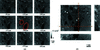Figure 2 (a) Sectional images and (b) expansion images of flat slabs of limestone from the Carnic Alps in the Carboniferous period. Red circles indicate two kinds of fusulinids: Daixina sp. (left) and Triticites cf. immutabilis (right).

For the feasibility observation of the film-shaped sample and the estimation of the spatial resolution, we observed some carbon paper used for gas diffusion layers (GDLs) of polymer electrolyte fuel cells (PEFCs). PEFCs have a sandwich structure consisting of a fuel electrode (anode), an electrolyte and an air electrode (cathode) held by bipolar plates. The performance of PEFCs mainly depends on the transport of water generated by the reaction of hydrogen and oxygen in the cathode GDLs, and therefore the visualization of the accumulation and transport behavior is indispensable. Fine three-dimensional observation of small demonstrative PEFCs was performed, and water in the GDLs was clearly visualized by absorption-contrast X-ray CT with low-energy X-rays (Koresawa & Utaka, 2014). For practical in situ observation with normal size PEFCs, high-energy X-rays with high transparency are required to transmit the thick bipolar plates sandwiching the GDLs. However, sensitivity decreases as X-ray energy increases, so fine observation to distinguish water and carbon cannot be performed using conventional X-ray imaging.

The sensitivity of phase-contrast X-ray imaging maintains a high level for high-energy X-rays, and fine observation can be performed. To date, water and ice contained in GDLs were clearly visualized by phase-contrast X-ray CT using a diffraction-enhanced imaging (DEI) method with 35 keV X-rays (Takeya et al., 2013). In addition to the above purpose, for a quantitative comparison of the density resolution with our previous results, GDLs were observed under almost the same measurement conditions, including X-ray energy, X-ray imager and X-ray doses.

Figs. 3(a) and 3(b) show the obtained sectional images of a carbon-paper film used for GDLs. The diameter of the film was 10 mm and the thickness was about 200 µm. The central area, which was a 4.5 mm square, was reconstructed by 1000 iterative calculations. The space interval of each image was 25 µm. Each single carbon fiber can be clearly visualized, and the overlapping order of each fiber can be identified by its shade. Fig. 3(c) shows the three-dimensional image obtained by volume rendering. The overlapping structure can be visualized more clearly. The average thickness of the fiber was about 30 µm (2.5 pixels) in the in-plane direction and 50 µm (4 pixels) in the sectional direction as shown in Fig. 3(b). The original thickness of the fiber was determined to be 10 µm (which is much smaller than the obtained thicknesses) by observation using scanning electron microscopy (SEM); the spatial resolution of the system was about 30 µm in the in-plane direction and 50 µm in the sectional direction. The spatial resolution in the sectional direction is approximately double that in the in-plane direction due to the inclined rotational angle of lamino­graphy.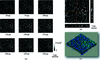Figure 3 (a) Sectional images, (b) expansion images and (c) a three-dimensional image of carbon paper used for fuel-cell batteries. Fiber structures were visualized clearly.

Fig. 4shows the sectional images obtained by the X-ray interferometric (XI) method (Fig. 4a) and DEI method (Fig. 4b) (Takeya et al., 2013). The dynamic range of density of the DEI method is much larger than that of the XI method, therefore normal CT of carbon paper can be performed. The similar distribution and overlapping structures of the carbon fibers can be seen. The DEI image was obtained at low temperature (−40°C), and ice areas were included in the image as shown in Fig. 4(b). The density of the carbon fiber was estimated to be 1.76 g cm−3 from the density of the background area (air) and ice. In addition, the density resolutions calculated from the standard deviation in the background area were estimated to be 0.004 and 0.05 g cm−3 for the XI and DEI methods, respectively. The ratio of each density resolution corresponded reasonably well with our previous result (Yoneyama et al., 2008). This result shows that the method enables us to perform fine observation of GDLs with high-energy X-rays, and it is expected that in situ (operand) observation of normal size PEFCs can be performed by utilizing its high transparency.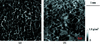Figure 4 Sectional images of carbon paper obtained by (a) XI and (b) the DEI method.

### 4. Conclusions

Detailed sectional images of a limestone slab and carbon paper were obtained using phase-contrast lamino­graphy with a crystal X-ray interferometer and high-energy X-rays. These results show that the imaging technique is a powerful tool to visualize the inner structure of the thick laminar sample with high-density resolution. Fine non-destructive three-dimensional observations of laminar samples packed by heavy metal such as a battery are expected with in situ conditions using high-energy X-rays. In addition, the combination of Zeff (effective atomic number) imaging (Yoneyama, Hyodo et al., 2013) provides a way to obtain three-dimensional elemental mapping of the laminar sample.

### Acknowledgements

We would like to show our appreciation to Dr Masahiro Ichida for the preparation of the flat slab and the fruitful discussions of fusulinids.

### Funding information

This study was carried out under Proposal Nos. 2009S2-006 and 2012-G044, which were approved by the High Energy Accelerator Research Organization.

### ReferencesJOURNAL OFSYNCHROTRONRADIATION